# Flow Expression Operators

You can use expressions and operators in your flow notation parameters to design robust interaction flows that behave in certain ways depending on their configuration.

This table includes examples of some common expressions that you can use with your flow.

 This list is a work in progress. We are working to improve and add to it on a regular basis. If an expression that you're looking for isn't currently listed, please check back on an ongoing basis.
Operator Description
Common Operators

count

`(count coll)`

Returns the number of items in the collection.

• Using (count nil) returns 0.
• Using (count string), where string is replaced with your string data, returns the length of the string.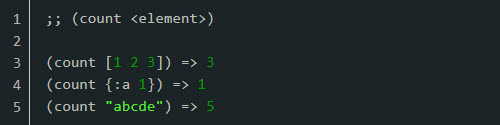String Operators

regex

`(regex patterns s)`

Checks if a string matches a pattern and returns a true response if it does and false if not.

• Any stray character is matched exactly.
• The Anyone character ('?') represents a single arbitrary character.
• Wildcards ('*') represent any number of arbitrary characters, including 0
• Character classes are enclosed in square brackets and may contain an arbitrary list of characters to match. If a character class begins with a ('!') or ('^') character, the class will be negated meaning it will only match characters absent from the class. Empty character classes such as ('[]'), ('[!]'), and ('[^]') match their representation, not their content.
• Trees match any of their branches. Trees have their boundary limited defined in curly brackets with the content within separated by commas. Empty trees such as ('{}'), ('{,}'), and ('{,,}') match their representation, not their content.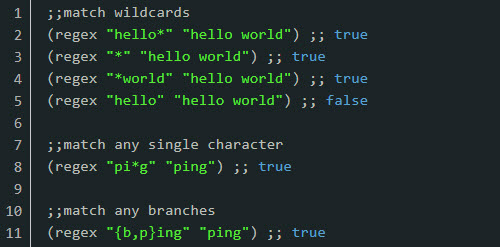str

`(str arg1 arg2 ....)`

Links all arguments to a string. The example provided represents the argument fields by the arg values.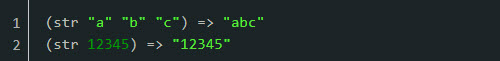subs

`(subs s start) `
`(subs s start end)`

Returns the substring of the value used for s beginning at start (inclusive), and ending at end (exclusive). The end defaults to the length of the string.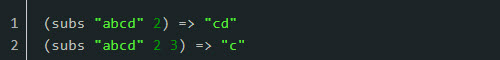join

`(join coll)`
`(join separator coll)`

Returns a string of all of the elements in a collection, as returned by (seq coll), separated by an operational separator.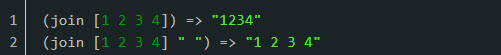escape

`(escape s cmap)`

Returns a new string, using cmap to escape each character (ch) from s as follows:

• If (cmap ch) is nil, append ch to the new string
• If (cmap ch) is not nil, append (str (cmap ch)) instead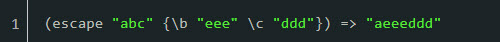split

`(split s separator)`
`(split s separator limit)`

Splits a string into a list of strings with a separator.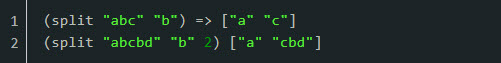split-lines

`(split-lines s)`

Splits the value for s on \n or \r\n.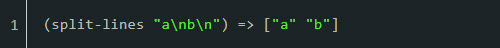replace

`(replace s match replacement)`

Replaces all instances of the value used for match with the value used for replacement in s.

• The match needs to be a string, not regex.replace-first

`(replace-first s match replacement)`

Replaces the first instance of the value used for match with the value used for replacement in s.re-quote-replacement

`(re-quote-replacement replacement)`

Given a replacement string that you wish to be a literal replacement for a pattern match in replace or replace-first, do the necessary escaping of special characters in the replacement.

reverse

`(reverse s)`

Returns the value for s with it's characters reversed.index-of

`(index-of s value)`
`(index-of s value from-index)`

Returns the index of the string or character value for s.

Optionally, you search forwards from from-index point or nil if not found.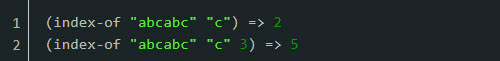last-index-of

`(last index-of s value)`
`(last-index-of s value from-index)`

Returns the last index of the string or character value for s.

Optionally, you can search backwards from from-index point or nil if not found.capitalize

`(capitalize s)`

Converts the first character of the string value to upper case and keeps all other characters lower case.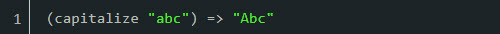lower-case

`(lower-case s)`

Converts the entire string to lower case characters.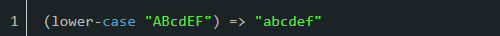upper-case

`(upper-case s)`

Converts the entire string to upper case characters.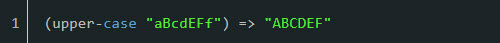trim

`(trim s)`

Removes any white space from both ends of the string.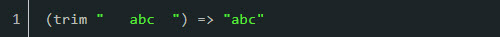trim-newline

`(trim-newline s)`

Removes any trailing newline (\n) or return (\r) characters from the string.triml

`(triml s)`

Removes any white space from the left side of the string.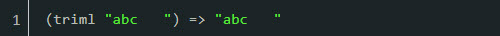trimr

`(trimr s)`

Removes any white space from the right side of the string.render

`(render s)`

Renders a mustache template with the current environment, using any assigned variables. If the variable is missing, the place holder will be resolved to an empty string as with the {{greeting}} variable below.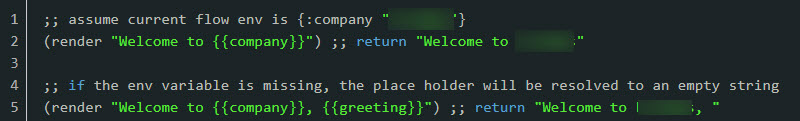string?

`(string? x)`

Returns as true if the value for x is a string.blank?

`(blank? s)`

Returns as true if the value for s is nil, empty, or contains only white space.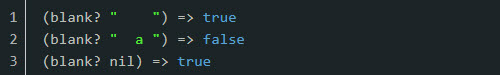starts-with?

`(starts-with? s substr)`

Returns as true if the string (s) starts with the substring (substr) provided.end-with?

`(ends-with? s substr)`

Returns as true if the string (s) ends with the substring (substr) provided.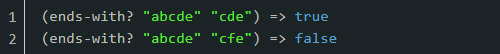includes?

`(includes? s substr)`

Returns as true if the string (s) includes the substring (substr) provided.Arithmetic Operators

+

`(+)`
`(+ x)`
`(+ x y) `
`(+ x y & more)`

Returns the sum of numbers.

• (+) returns 0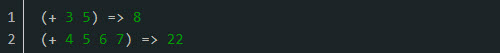-

`(- x)`
`(- x y)`
`(- x y & more)`

Subtracts the y's from x and returns the result.

• If no y's are supplied, returns the negation of x*

`(*)`
`(* x)`
`(* x y)`
`(* x y & more)`

Returns the product of the numbers.

• (*) returns a value of 1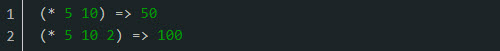/

`(/ x)`
`(/ x y)`
`(/ x y & more)`

Returns the numerator divided by all of the denominators.

• If no denominators are supplied, returns 1/numeratorquot

`(quot num div)`

Returns the quotient of dividing the numerator by the denominator.

• (quot m n) is the value of m/n, rounded towards 0 to the nearest integer.
• m, n do not need to be integers.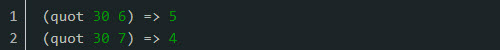rem

`(rem num div)`

Returns the remainder of dividing the numerator by the denominator.

• rem and mod are commonly used to get the remainder. mod means Gaussian mod, sot he result is always non-negative. Don't confuse it with ANCI C's % operator which, despite being pronounced "mod", actually implements rem as with (rem -10 3) -1.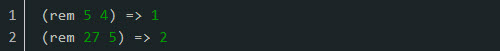mod

`(mod num div)`

Returns the modulus of num and div.

• Truncates towards negative infinity.
• It's defined as the amount by which a number exceeds the largest integer multiple of the divisor that is not greater than that number. The largest integer multiple of 5 not greater than -2 is 5 * -1 = -5. The amount by which -2 exceeds -5 is 3.inc

`(inc x)`

Returns a number one greater than num.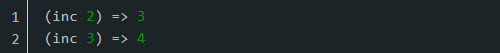dec

`(dec x)`

Returns a number one less than the num.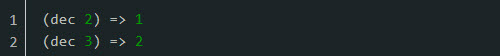max

`(max x) `
`(max x y) `
`(max x y & more)`

Returns the greatest of the nums.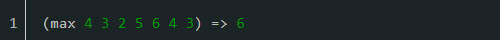min

`(max x) `
`(max x y) `
`(max x y & more)`

Returns the least of the nums.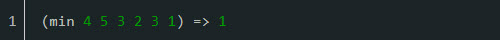+'

`(+')`
`(+' x)`
`(+' x y)`
`(+' x y & more)`

Returns the sum of the numbs.

• (+) returns 0.
• Supports arbitrary precision.

-'

`(-' x)`
`(-' x y)`
`(-' x y & more)`

Subtracts the y's from x and returns the result.

• If no y's are supplied, returns the negation of x.
• Supports arbitrary precision.

*'

`(*')`
`(*' x)`
`(*' x y)`
`(*' x y & more)`

Returns the product of the numbers.

• (*) returns a value of 1.
• Supports arbitrary precision.

inc'

`(inc' x)`

Returns a number one greater than num.

• Supports arbitrary precision.

dec'

`(dec' x)`

Returns a number one less than the num.

• Supports arbitrary precision.
Math Operators

rand

`(rand)`
`(rand n)`

Returns a random floating point number between 0 (inclusive) and n (default 1) (exclusive).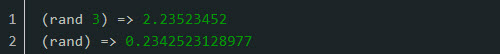rand-int

`(rand-int n)`

Returns a random integer between 0 (inclusive) and n (exclusive).abs

`(abs x)`

Returns the absolute value of x.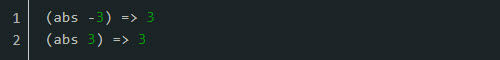floor

`(floor x)`

Returns x, rounded down to the nearest integer.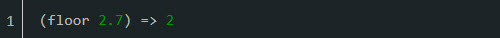ceiling

`(ceiling x)`

Returns x, rounded up to the nearest integer.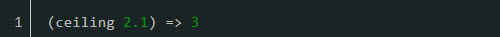round

`(round x y)`

If the fractional portion of x is 0.5 or greater, the argument is rounded up to the nearest integer. If the fractional portion of x is less than 0.5, the argument is rounded down to the nearest integer.

• If y is specified then it will round x to the number of decimal places specified.
• If y=0 then it will round to the nearest integer.Number Comparison Operators

zero?

`(zero? x)`

Returns true if num is zero, otherwise it's false.pos?

`(pos? x)`

Returns true if num is greater than zero, otherwise it's false.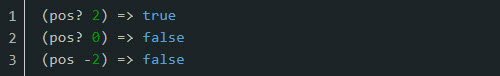neg?

`(nex? x)`

Returns true if num is less than zero, otherwise it's false.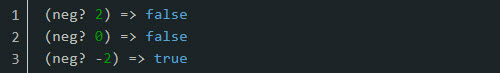even?

`(even? n)`

Returns true if n is even, otherwise it's false.

• Throws an exception if n is not an integer.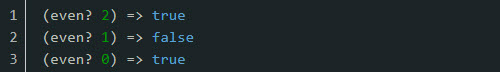odd?

`(odd? n)`

Returns true if n is odd, otherwise it's false.

• Throws an exception if n is not an integer.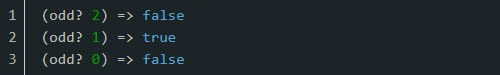number?

`(number? x)`

Returns true if x is a number.rational?

`(rational? n)`

Returns true if n is a rational number, otherwise it's false.

integer?

`(integer? n)`

Returns true if n is an integer, otherwise it's false.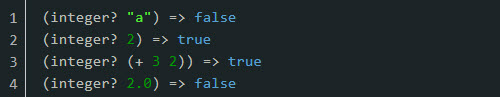ratio?

`(ratio? n)`

Returns true if n as a ratio, otherwise it's false.

decimal?

`(decimal? n)`

Returns true if n is a BigDecimal, otherwise it's false.

float?

`(float? n)`
Returns true if n is a floating point number, otherwise it's false.

sort

`(sort coll)`
`(sort comp coll)`
Returns a sorted sequence of the items in the collection (coll). It checks to see if a string matches on a pattern and returns true/false.

sort-by

`(sort-by key coll)`
`(sort-by key comp coll)`
Returns a sorted sequence of the items in the collection (coll), where the sort order is determined by comparing the key value of each item.
UUIDs

as-uuid

The as-uuid function will allow the conversion (and also verification) of a string to a uuid object. If the passed object is already a uuid, the same object will be returned.uuid-v1

The uuid-v1 function will return a new v1 UUID. The value returned will be a UUID, not a string.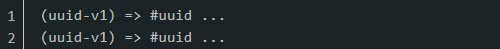uuidable?

`(uuidable? x)`

Returns true if x can be coerced into a uuid, otherwise it's false.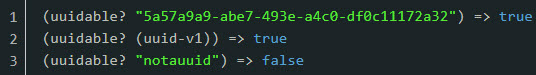uuid?

`(uuid? x) `

Returns true if x is a uuid, otherwise it's false.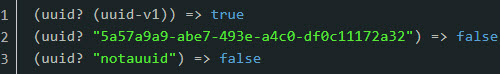Phone Number Operators

phone-number

`(phone-number number)`
`(phone-number number locale)`

Parses a string telephone number into a map of different formats.

• Adding the locale parses a string number, using locale, into a map of different formats.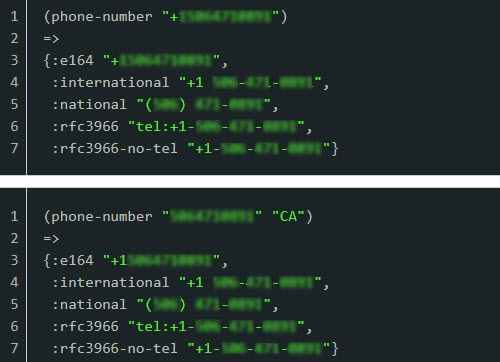Date and Time Operators

days-this-month

`(days-this-month)`

Returns the number of days in the current month.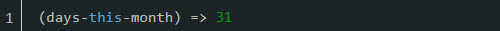days-in-month

`(days-in-month date)`

Returns the number of days in the month of a specified date.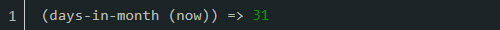now

`(now)`
Returns the current date and time.

today

`(today)`

Returns today's date.

• Does not include the time.

today-at-midnight

`(today-at-midnight)`
Returns the date and time as of midnight today.

yesterday

`(yesterday)`

Returns the date for yesterday.

• Does not include a time.

tomorrow

`(tomorrow)`

Returns the date for tomorrow.

• Does not include a time.

ago

`(ago t)`
Returns the date and time as it was t seconds/minutes/hours/days/months/years ago.

from-now

`(from-now t)`
Returns the date and time that it will be in t seconds/minutes/hours/days/months/years from now.

second

`(second t)`
Returns the seconds portion of a date specified by t.

minute

`(minute t)`
Returns the minutes portion of a date specified by t.

hour

`(hour t)`
Returns the hour portion of a date specified by t.

day

`(day t)`
Returns the day of the month in a date specified by t.

month

`(month t)`

Returns the month portion of a date, specified by t, as number.

• For example, March would return as 3.

quarter

`(quarter t start)`

Returns the quarter of a date along with the year. The start is the number of the month in the year that you want the quarters to start in. If no start is specified, then January (1) is used as the start of the quarters.

For example, if the start = 1 or isn't specified, returns the year along with:

• 1 if the month is January, February, or March
• 2 if the month is April, May, or June
• 3 if the month is July, August, or September
• 4 if the month is October, November, or December.

Alternatively, if the start = 5, returns the year along with:

• 1 if the month is May, June, or July
• 2 if the month is August, September, or October
• 3 if the month is November, December, or January
• 4 if the month is February, March, or Aprilyear

`(year t)`
Returns the year portion of a date specified by t.

day-of-week

`(day-of-week t)`
Returns the numeric value for the day of the week for a given date (t), between 1 and 7, where Sunday is considered 1 and the first day of the week.

week-of-year

`(week-of-year t)`
Returns the number value for the week of the year for a given date (t).

end-of-month

`(end-of-month t)`
Returns the numeric value for the last day of the month (t).

beginning-of-month

`(beginning-of-month t)`
Returns the numeric value for the beginning of the month (t).

at-midnight

`(at-midnight t)`
Returns the date/time at midnight for a given date (t).

ampm

`(ampm t)`

Returns am if the current time is between 00:00 inclusive and 12:00 exclusive. Returns pm if the current time is between 12:00 inclusive and 00:00 exclusive.

• 11:59:59 would return am, while 23:59:59 would return pm.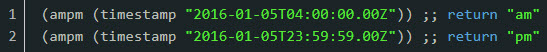timezone-offset

`(timezone-offset d)`
`(timezone-ofset d d2)`

Returns the offset between a date (d) and another (d2).

• If no other date (d2) is provided, uses UTC date.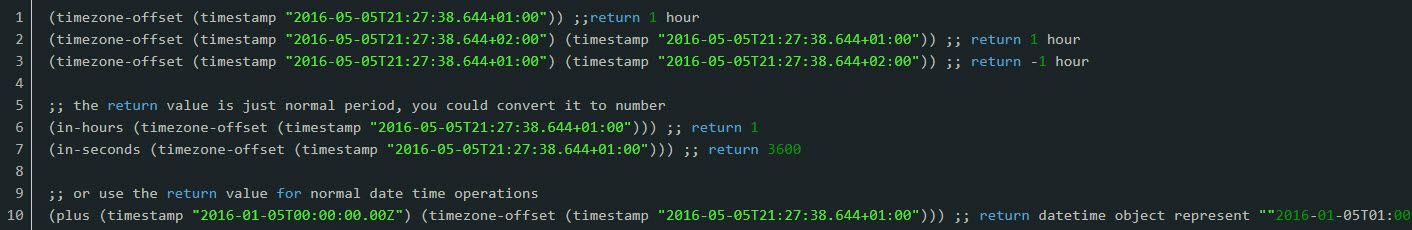interval

`(interval date1 date2)`

Returns the interval between date1 and date2.

• date1 must be earlier than or the same time as date2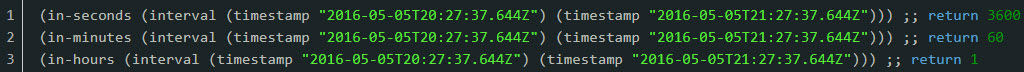Date and Time Periods

seconds

`(seconds t)`
Returns a date considered to be that many seconds.

minutes

`(mintues t)`
Returns a date considered to be that many minutes.

hours

`(hours t)`
Returns a date considered to be that many hours.

days

`(days t)`
Returns a date considred to be that many days.

months

`(months t)`
Returns a date considered to be that many months.

quarters

`(quarters t)`

Returns a date considered to be that many quarters.

• A quarter is considered to be a 3 month period of a year.

years

`(years t)`
Returns a date considered to be that many years.

in-seconds

`(in-seconds p)`

Returns the number of seconds in a given period.

• See in-years for an example.

in-days

`(in-days p)`

Returns the number of days in a given period.

• See in-years for an example.

in-weeks

`(in-weeks p)`

Returns the number of weeks in a given period.

• See in-years for an example.

in-months

`(in-months p)`

Returns the number of months in a given period.

• See in-years for an example.

in-years

`(in-years p)`

Returns the number of years in a given period.Date and Time Predicates and Comparators

after?

`(afetr? a b)`

Returns true if a comes after b, otherwise it is false.

• For example, (after? (tomorrow) (now)) will return true.

before?

`(before? a c)`

Returns true if a occurs after b, otherwise it is false.

• For example, (before? (yesterday) (now)) will return true.

within?

`(within? a b c)`

Returns true if b occurs after a and before c, otherwise it is false.

• For example, (within? (yesterday) (tomorrow) (now)) will return true.

earliest

`(earliest a b c & more)`

Returns the earliest date/time in a given list of date/times.

• For example, (earliest (yesterday) (now)) will return the date for yesterday.

latest

`(latest a b c & more)`

Returns the latest date/time in a given list of date/times.

• For example, (latest (yesterday) (now)) will return the date for now.
Date and Time Conversion

unix-timestamp

`(unix-timestamp s)`

Converts s to an unix timestamp.

• s could be a datetime string or datetime object.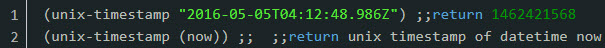timestamp

`(timestamp s)`

Converts s to a datetime object.

• s could be a datetime string or datetime object.Date and Time Formatting

format-date

`(format-date t)`
`(format-date formatter t)`

Given a date, returns the date in a specified format.Maps

get

`(get <map> <key>)`

Allows for the retrieval of a key from a map.

• The key will always be cast to a keyword.
• You can also use the dot syntax instead of the get command.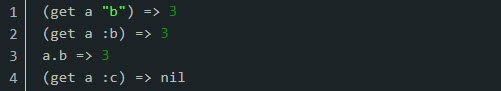get-in

`(get <map> [<key>*])`

• As with get, you can also use dot notation.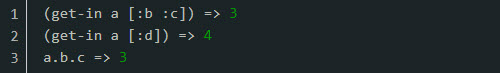assoc

`(get <map> <<key> <value>>*)`

Allows you to add a key and a value to a map.

• If the key already exists in the map, the original value will be overwritten.assoc-in

`(assoc <map> <<key> <value>*)`

Allows you to add a key and a value to a map.

• If the key already exists in the map, the original value will be overwritten.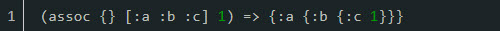select-keys

`(select-keys <map> <key>*)`

Returns a map which only contains the keys specified.

• If any of the keys do not exist in the provided map, they will not be present in the resulting map.remove-keys

`(remove-keys <map> <key>*)`

Removes the provided keys from the provided map.

• Keys will automatically be converted to keywords.

filter-vals

`(filter-vals <map> <predicate>)`

Specifies a predicate to test all the values of a map with.

• All values which result in true will be kept, and all false values will be discarded along with their keys.
• When specifying the predicate fn in the placeholder for the value is the _ symbol. While the predicate fn can be arbitrarily complex, the _ charachter must appear at the top level.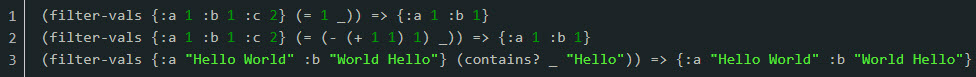Lists

`(add <list> <value>*)`

Adds a value to the end of a list.remove

`(remove <list> <value>*)`

Removes all occurrences of a value from a list.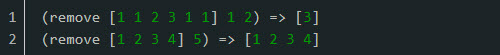contains?

`(contains <list> <value>)`

Returns true if a list contains the value specified, otherwise it is false.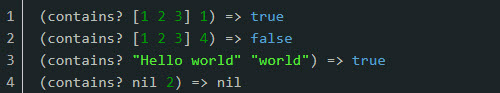Control

if

`(if <predicate> <true> [<false>])`

The if command is the basic control structure, allowing for true and false (else) paths.

• If no false path is provided, it will evaluate to nil.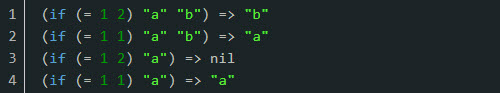cond

`(cond <<predicate> <value>>* [:else <value>])`

The cond command is an extension of if and allows for a more compact expression of multiple predicates.

• Optionally, an else value can be provided by adding the predicate :else at the last predicate/value pair.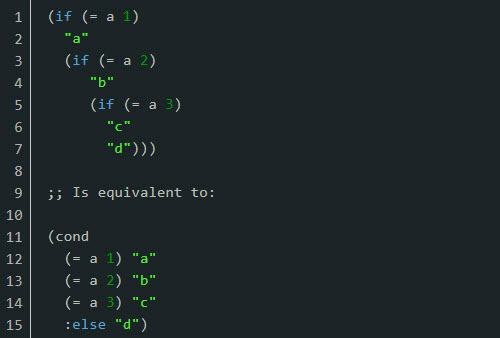find

`(find <list> <lookup-key> <value>)`

Allows for searching a list of maps for the first element that equals the provided value.

• In it's current form, find is only useful for a list of maps.Comparator Operators
= Returns true if x equals y, false if not.
!= Negation of =.
not Returns true if x is logical false, false if not.
not= Negation of =.
> Returns non-nil if numbers are in monotonically decreasing order, otherwise it's false.
>= Returns non-nil if numbers are in monotonically non-increasing order, otherwise it's false.
< Returns non-nil if numbers are in monotonically increasing orde, otherwise it's false.
<= Returns non-nil if numbers are in monotonically non-decreasing order, otherwise it's false.
and

Evaluates expression one at a time, from left to right.

• If a form returns logical false (nil or false), and returns that value and doesn't evaluate any of the other expressions, otherwise it returns the value of the last expression.
• (and) returns true.
or

Evaluates expression one at a time, from left to right.

• If a form returns a logical true value, or returns that value and doesn't evaluate any of the other expressions, otherwise it returns the value of the last expression.
• (or) returns nil.